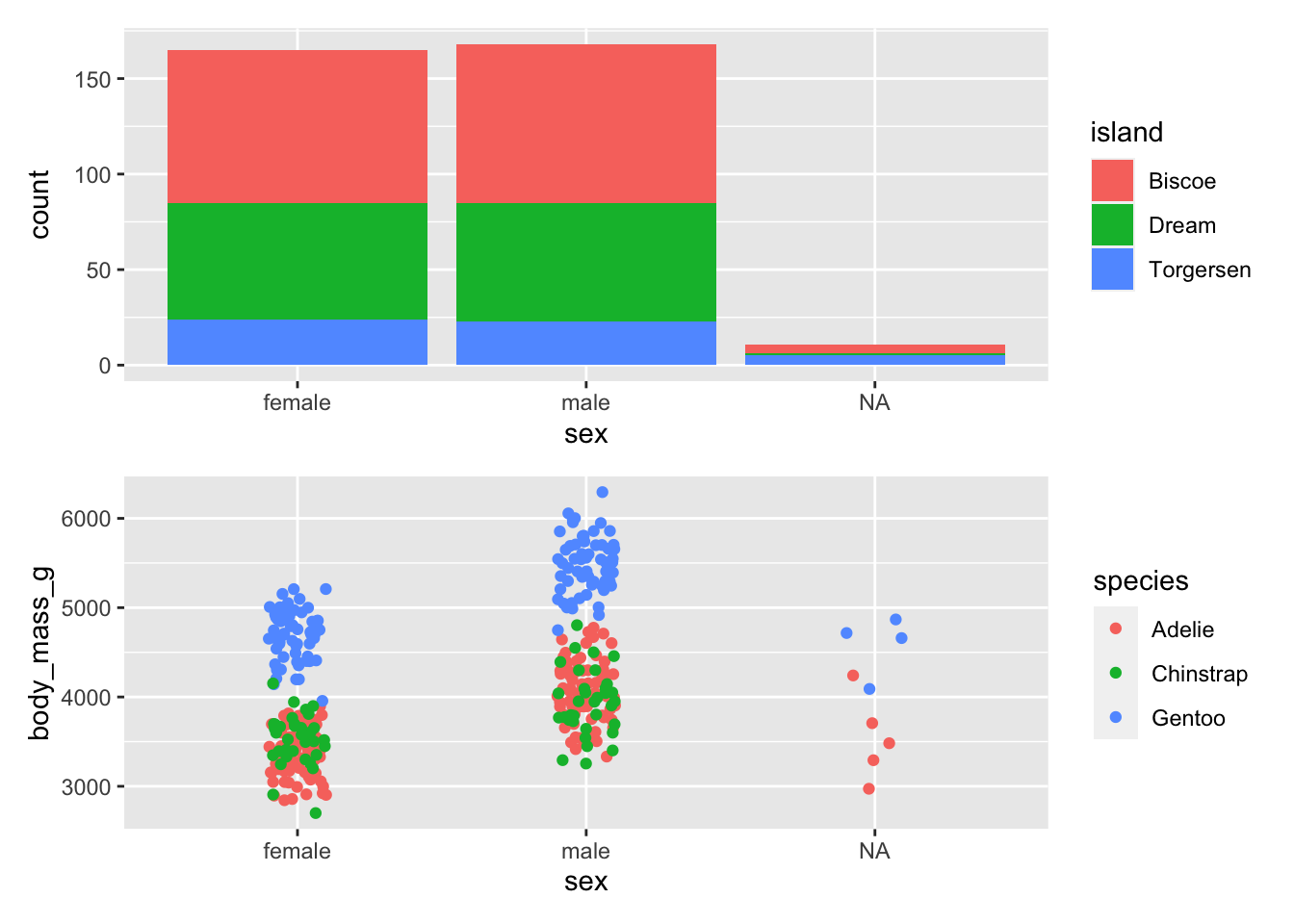## 16.5 More than one plot

There are several ways to place more than one plot side by side. One of the easiest is to use patchwork.

Install

install.packages("patchwork")

library(patchwork)

This time we store our plots as variables

barGraph <- ggplot(data = penguins, aes(x = sex, fill = island)) +
geom_bar()

dotPlot <- ggplot(data = penguins, aes(x = sex, y = body_mass_g, colour = species)) +
geom_jitter(width = 0.1)

Then patchwork will arrange them

barGraph + dotPlotbarGraph / dotPlotThere are many ways in which patchwork can arrange plots. See the chapter Arranging Plots in ggplot2: Elegant Graphics for Data Analysis for more complex examples.